##### Algebra I Workbook For Dummies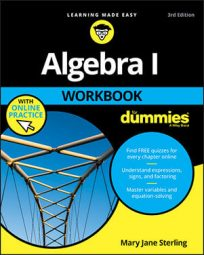In algebra, an improper fraction is one where the numerator (the number on the top of the fraction) has a value greater than or equal to the denominator (the number on the bottom of the fraction) — the fraction is top heavy.

Improper fractions can be written as mixed numbers or whole numbers — and vice versa. For example,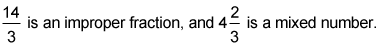## Practice questions

1. Change the mixed number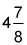to an improper fraction.

2. Change the improper fraction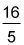to a mixed number.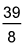To change a mixed number to an improper fraction, you need to multiply the whole number times the denominator and add the numerator. This result goes in the numerator of a fraction that has the original denominator still in the denominator.

So, do the following math: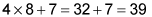This means that the improper fraction is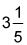To change an improper fraction to a mixed number, you need to divide the numerator by the denominator and write the remainder in the numerator of the new fraction.

In this example, to change the improper fraction 16/5 to a mixed number, do the following: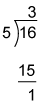Think of breaking up the fraction into two pieces: One piece is the whole number 3, and the other is the remainder as a fraction, 1/5.